PROC VARCLUS Statement
PROC VARCLUS <options> ;

The PROC VARCLUS statement starts PROC VARCLUS. By default, VARCLUS clusters the numeric variables in the most recently created SAS data set, starting with one cluster and splitting clusters until all clusters have at most one eigenvalue greater than one.

Table 96.1 summarizes the options available in the PROC VARCLUS statement.

Table 96.1 Options Available in the PROC VARCLUS Statement

Option

Description

Data Sets

Specifies the input SAS data set

Specifies the output SAS data set to contain statistics

Specifies the output SAS data set for use with PROC TREE

Input Data Processing

Uses the covariance matrix instead of the correlation matrix

Omits the intercept

Specifies the divisor for variances

Number of Clusters

Specifies the maximum number of clusters

Specifies the minimum number of clusters

Specifies the maximum second eigenvalue in a cluster

Specifies the minimum proportion of variance explained by a cluster component

Clustering Methods

Uses centroid components instead of principal components

Clusters hierarchically

Specifies the initialization method

Specifies the maximum iterations during the alternating least squares phase

Specifies the maximum iterations during the search phase

Performs a multiple group component analysis

Specifies the random number seed

Control Displayed Output

Displays the correlation matrix

Suppresses displayed output

Specifies ODS Graphics details

Suppresses display of large matrices

Displays means and standard deviations

Suppresses all default displayed output except the final summary table

Displays the cluster to which each variable is assigned during the iterations

VARCLUS chooses which cluster to split based on the MAXEIGEN= and PROPORTION= options.

1. If you specify either or both of these two options, then only the specified options affect the choice of the cluster to split.

2. If you specify neither of these options, the criterion for choice of cluster to split depends on the CENTROID option:

1. If you specify CENTROID, VARCLUS splits the cluster with the smallest percentage of variation explained by its cluster component, as if you had specified the PROPORTION= option.

2. If you do not specify CENTROID, VARCLUS splits the cluster with the largest eigenvalue associated with the second principal component, as if you had specified the MAXEIGEN= option.

The final number of clusters is controlled by three options: MAXCLUSTERS=, MAXEIGEN=, and PROPORTION=.

1. If you specify any of these three options, then only the options you specify affect the final number of clusters.

2. If you specify none of these options, VARCLUS continues to split clusters until the default splitting criterion is satisfied. The default splitting criterion depends on the CENTROID option:

1. If you specify CENTROID, the default splitting criterion is PROPORTION=0.75.

2. If you do not specify CENTROID, splitting is based on the MAXEIGEN= criterion, with a default depending on the COVARIANCE option:

1. For analyzing a correlation matrix (no COVARIANCE option), the default value for MAXEIGEN= is one.

2. For analyzing a covariance matrix (using the COVARIANCE option), the default value for MAXEIGEN= is the average variance of the variables being clustered.

VARCLUS continues to split clusters until any of the following conditions holds:

• The number of cluster equals the value specified for MAXCLUSTERS=.

• No cluster qualifies for splitting according to the MAXEIGEN= or PROPORTION= criterion.

• A cluster was chosen for splitting, but after iteratively reassigning variables to clusters, one of the cluster has no members.

The following list gives details about the options.

CENTROID

uses centroid components rather than principal components. You should specify centroid components if you want the cluster components to be unweighted averages of the standardized variables (the default) or the unstandardized variables (if you specify the COVARIANCE option). It is possible to obtain locally optimal clusterings in which a variable is not assigned to the cluster component with which it has the highest squared correlation. You cannot specify both the CENTROID and MAXEIGEN= options.

CORR
C

displays the correlation matrix.

COVARIANCE
COV

analyzes the covariance matrix instead of the correlation matrix. The COVARIANCE option causes variables with a large variance to have more effect on the cluster components than variables with a small variance.

DATA=SAS-data-set

specifies the input data set to be analyzed. The data set can be an ordinary SAS data set or TYPE=CORR, UCORR, COV, UCOV, FACTOR, or SSCP. If you do not specify the DATA= option, the most recently created SAS data set is used. See Appendix A, Special SAS Data Sets, for more information about types of SAS data sets.

HIERARCHY
HI

requires the clusters at different levels to maintain a hierarchical structure. To draw a tree diagram, enable ODS Graphics or use the OUTTREE= option and the TREE procedure.

INITIAL=GROUP
INITIAL=INPUT
INITIAL=RANDOM
INITIAL=SEED

specifies the method for initializing the clusters. If the INITIAL= option is omitted and the MINCLUSTERS= option is greater than 1, the initial cluster components are obtained by extracting the required number of principal components and performing an orthoblique rotation (raw quartimax rotation on the eigenvectors; Harris and Kaiser 1964). The following list describes the values for the INITIAL= option:

GROUP

obtains the cluster membership of each variable from an observation in the DATA= data set where the _TYPE_ variable has a value of 'GROUP'. In this observation, the variables to be clustered must each have an integer value ranging from one to the number of clusters. You can use this option only if the DATA= data set is a TYPE=CORR, UCORR, COV, UCOV, or FACTOR data set. You can use a data set created either by a previous run of PROC VARCLUS or in a DATA step.

INPUT

obtains scoring coefficients for the cluster components from observations in the DATA= data set where the _TYPE_ variable has a value of 'SCORE'. You can use this option only if the DATA= data set is a TYPE=CORR, UCORR, COV, UCOV, or FACTOR data set. You can use scoring coefficients from the FACTOR procedure or a previous run of PROC VARCLUS, or you can enter other coefficients in a DATA step.

RANDOM

assigns variables randomly to clusters.

SEED

initializes each cluster component to be one of the variables named in the SEED statement. Each variable listed in the SEED statement becomes the sole member of a cluster, and the other variables are initially unassigned. If you do not specify the SEED statement, the first MINCLUSTERS= variables in the VAR statement are used as seeds.

MAXCLUSTERS=n
MAXC=n

specifies the largest number of clusters desired. The default value is the number of variables. VARCLUS stops splitting clusters after the number of clusters reaches the value of the MAXCLUSTERS= option, regardless of what other splitting options are specified.

MAXEIGEN=n

specifies that when choosing a cluster to split, VARCLUS should choose the cluster with the largest second eigenvalue, provided that its second eigenvalue is greater than the MAXEIGEN= value. The MAXEIGEN= option cannot be used with the CENTROID or MULTIPLEGROUP options.

If you do not specify MAXEIGEN=, the default behavior depends on other options as follows:

• If you specify PROPORTION=, CENTROID, or MULTIPLEGROUP, cluster splitting does not depend on the second eigenvalue.

• Otherwise, if you specify MAXCLUSTERS=, the default value for MAXEIGEN= is zero.

• Otherwise, the default value for MAXEIGEN= is either 1.0 if the correlation matrix is analyzed or the average variance if the COVARIANCE option is specified.

If you specify both MAXEIGEN= and MAXCLUSTERS=, the number of clusters will never exceed the value of the MAXCLUSTERS= option.

If you specify both MAXEIGEN= and PROPORTION=, VARCLUS first looks for a cluster to split based on the MAXEIGEN= criterion. If no cluster meets that criterion, VARCLUS then looks for a cluster to split based on the PROPORTION= criterion.

MAXITER=n

specifies the maximum number of iterations during the NCS phase. The default value is 1 if you specify the CENTROID option; the default is 10 otherwise.

MAXSEARCH=n

specifies the maximum number of iterations during the search phase. The default is 1,000 divided by the number of variables.

MINCLUSTERS=n
MINC=n

specifies the smallest number of clusters desired. The default value is 2 for INITIAL=RANDOM or INITIAL=SEED; otherwise, VARCLUS begins with one cluster and tries to split it in accordance with the PROPORTION= option or the MAXEIGEN= option or both.

MULTIPLEGROUP
MG

performs a multiple group component analysis (Harman; 1976). You specify which variables belong to which clusters. No clusters are split, and no variables are reassigned to a different cluster. The input data set must be TYPE=CORR, UCORR, COV, UCOV, FACTOR, or SSCP and must contain an observation with _TYPE_='GROUP' that defines the variable groups. Specifying the MULTIPLEGROUP option is equivalent to specifying all of the following options: INITIAL=GROUP, MINC=1, MAXITER=0, MAXSEARCH=0, PROPORTION=0, and MAXEIGEN=large number.

NOINT

requests that no intercept be used; covariances or correlations are not corrected for the mean. If you specify the NOINT option, the OUTSTAT= data set is TYPE=UCORR.

NOPRINT

suppresses displayed output. This option temporarily disables the Output Delivery System (ODS). For more information, see Chapter 20, Using the Output Delivery System.

OUTSTAT=SAS-data-set

creates an output data set to contain statistics including means, standard deviations, correlations, cluster scoring coefficients, and the cluster structure. If you want to create a permanent SAS data set, you must specify a two-level name. The OUTSTAT= data set is TYPE=UCORR if the NOINT option is specified. For more information about permanent SAS data sets, see "SAS Files" and "DATA Step Concepts" in SAS Language Reference: Concepts. For information about types of SAS data sets, see Appendix A, Special SAS Data Sets.

OUTTREE=SAS-data-set

creates an output data set to contain information about the tree structure that can be used by the TREE procedure to display a tree diagram. The OUTTREE= option implies the HIERARCHY option. See Example 96.1 for use of the OUTTREE= option. If you want to create a permanent SAS data set, you must specify a two-level name. For more information about permanent SAS data sets, see "SAS Files" and "DATA Step Concepts" in SAS Language Reference: Concepts.

PLOTS <(global-plot-options)> <= plot-request >
PLOTS <(global-plot-options)> <= (plot-request <... plot-request >)>

controls the plots produced through ODS Graphics.

ODS Graphics must be enabled before requesting plots. For example:

```ods graphics on;

proc varclus plots=dendrogram(height=ncl);
run;

ods graphics off;
```

For more information about enabling and disabling ODS Graphics, see the section Enabling and Disabling ODS Graphics in Chapter 21, Statistical Graphics Using ODS.

By default, PROC VARCLUS produces a dendrogram.

The global-plot-options, UNPACK and ONLY, that are commonly used in the PLOTS= option in other procedures are accepted in PROC VARCLUS, but they currently have no effect since PROC VARCLUS produces only a dendrogram.

The following plot-requests can be specified:

ALL

produces all plots, which for PROC VARCLUS is only a dendrogram.

MAXPOINTS=n
MAXPTS=n

suppresses the dendrogram when the number of variables (clusters) exceeds the n value. This prevents an unreadable plot from being produced. The default is MAXPOINTS=200.

DENDROGRAM <( dendrogram-options )>

requests a dendrogram and specifies dendrogram-options.

Unlike most graphs, the size of the dendrogram can vary as a function of the number of objects that appear in the dendrogram. You can specify the following dendrogram-options to control the size and appearance of the dendrogram:

COMPUTEHEIGHT=a b
CH=a b

specifies the constants for computing the height of the dendrogram. For n points being clustered, intercept a, and slope b, the height is based in part on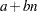. For a horizontal dendrogram, the default (given in pixels) is COMPUTEHEIGHT=100 12, the default height in pixels is max(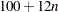, 480), the default height in inches is max(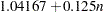, 5), and the default height in centimeters is max(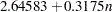, 12.7). For a vertical dendrogram, the default height is 480 pixels. The default unit is pixels, and you can use the UNIT= dendrogram-option to change the unit to inches or centimeters for this option. Inches equals pixels divided by 96, and centimeters equals inches times 2.54.

COMPUTEWIDTH=a b
CW=a b

specifies the constants for computing the width of the dendrogram. For n points being clustered, intercept a, and slope b, the width is based in part on. For a vertical dendrogram, the default (given in pixels) is COMPUTEWIDTH=100 12, the default width in pixels is max(, 640), the default width in inches is max(, 6.66667), and the default width in centimeters is max(, 16.933). For a horizontal dendrogram, the default width is 640 pixels. The default unit is pixels, and you can use the UNIT= dendrogram-option to change the unit to inches or centimeters for this option. Inches equals pixels divided by 96, and centimeters equals inches times 2.54.

HEIGHT=PROPORTION |  NCL  |  VAREXP
H=P |  N |  V

specifies the method for drawing the height of the dendrogram. HEIGHT=PROPORTION is the default.

HEIGHT=PROPORTION specifies that the total proportion of variance explained by the clusters at the current level of the tree is used.

HEIGHT=NCL specifies that the number of clusters is used.

HEIGHT=VAREXP specifies that the total variance explained by the clusters at the current level of the tree is used.

HORIZONTAL  |  VERTICAL

specifies either a horizontal dendrogram with the objects on the vertical axis (HORIZONTAL) or a vertical dendrogram with the objects on the horizontal axis (VERTICAL). The default is HORIZONTAL.

SETHEIGHT=height
SH=height

specifies the height of the dendrogram. By default, the height is based on the COMPUTEHEIGHT= option. The default unit is pixels, and you can use the UNIT= dendrogram-option to change the unit to inches or centimeters for this dendrogram-option.

SETWIDTH=width
SW=width

specifies the width of the dendrogram. By default, the width is based on the COMPUTEWIDTH= option. The default unit is pixels, and you can use the UNIT= dendrogram-option to change the unit to inches or centimeters for this dendrogram-option.

UNIT=PX  |  IN  |  CM

specifies the unit (pixels, inches, or centimeters) for the SETHEIGHT=, SETWIDTH=, COMPUTEHEIGHT=, and COMPUTEWIDTH= dendrogram-options.

NONE

suppresses all plots.

The names of the graphs that PROC VARCLUS generates are listed in Table 96.4, along with the required statements and options.

PROPORTION=n
PERCENT=n

specifies that when choosing a cluster to split, VARCLUS should choose the cluster with the smallest proportion of variation explained, provided that the proportion of variation explained is less than the PROPORTION= value. Values greater than 1.0 are considered to be percentages, so PROPORTION=0.75 and PERCENT=75 are equivalent.

However, if you specify both MAXEIGEN= and PROPORTION=, VARCLUS first looks for a cluster to split based on the MAXEIGEN= criterion. If no cluster meets that criterion, VARCLUS then looks for a cluster to split based on the PROPORTION= criterion.

If you do not specify PROPORTION=, the default behavior depends on other options as follows:

• If you specify MAXEIGEN=, cluster splitting does not depend on the proportion of variation explained.

• Otherwise, if you specify CENTROID and MAXCLUSTERS=, the default value for PROPORTION= is 1.0.

• Otherwise, if you specify CENTROID without MAXCLUSTERS=, the default value is PROPORTION=0.75 or PERCENT=75.

• Otherwise, cluster splitting does not depend on the proportion of variation explained.

If you specify both PROPORTION= and MAXCLUSTERS=, the number of clusters will never exceed the value of the MAXCLUSTERS= option.

RANDOM=n

specifies a positive integer as a starting value for use with REPLACE=RANDOM. If you do not specify the RANDOM= option, the time of day is used to initialize the pseudorandom number sequence.

SHORT

suppresses display of the cluster structure, scoring coefficient, and intercluster correlation matrices.

SIMPLE
S

displays means and standard deviations.

SUMMARY

suppresses all default displayed output except the final summary table.

TRACE

displays the cluster to which each variable is assigned during the iterations.

VARDEF=DF
VARDEF=N
VARDEF=WDF
VARDEF=WEIGHT | WGT

specifies the divisor to be used in the calculation of variances and covariances. The default value is VARDEF=DF. The values and associated divisors are displayed in the following table.

 Value Divisor Formula DF Degrees of freedom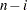N Number of observations n WDF Sum of weights minus one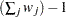WEIGHT | WGT Sum of weights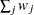In the preceding table,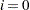if the NOINT option is specified, and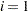otherwise.